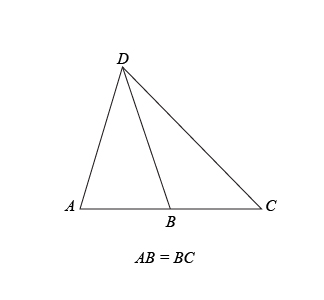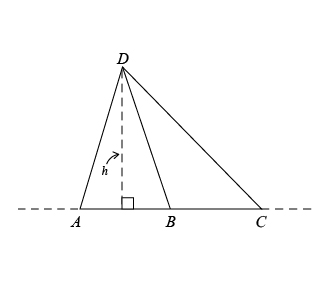# The area of △ABD The area of △BCD\$\$AB=BC\$\$

 Quantity A \$\;\;\;\;\;\;\;\;\;\;\;\;\;\;\;\;\;\;\;\;\;\;\;\;\;\;\;\;\;\;\;\;\;\;\;\;\;\;\;\;\;\;\;\;\;\;\;\;\;\;\;\;\;\;\;\;\;\;\;\;\;\$ Quantity B \$\;\;\;\;\;\;\;\;\;\;\;\;\;\;\;\;\;\;\;\;\;\;\;\;\;\;\;\;\;\;\;\;\;\;\;\;\;\;\;\;\;\;\;\;\;\;\;\;\;\;\;\;\;\;\;\;\;\;\;\;\;\$ The area of \$△ABD\$ \$\;\;\;\;\;\;\;\;\;\;\;\;\;\;\;\;\;\;\;\;\;\;\;\;\;\;\;\;\;\;\;\;\;\;\;\;\;\;\;\;\;\;\;\;\;\;\;\;\;\;\;\;\;\;\;\;\;\;\;\;\;\$ The area of \$△BCD\$
1. Quantity A is greater.
2. Quantity B is greater.
3. The two quantities are equal.
4. The relationship cannot be determined from the information given.

So, you were trying to be a good test taker and practice for the GRE with PowerPrep online. Buuuut then you had some questions about the quant section—specifically question 2 of the second Quantitative section of Practice Test 1. Those questions testing our knowledge of Triangles can be kind of tricky, but never fear, PrepScholar has got your back!

## Survey the Question

Let’s search the problem for clues as to what it will be testing, as this will help shift our minds to think about what type of math knowledge we’ll use to solve this question. Pay attention to any words that sound math-specific and anything special about what the numbers look like, and mark them on your paper.

We want to compare the areas of two triangles, so naturally we will draw upon our knowledge of the Triangles math skill for the GRE. Let’s keep what we’ve learned about this skill at the tip of our minds as we approach this question.

## What Do We Know?

Let’s carefully read through the question and make a list of the things that we know.

1. We’re comparing the area of two triangles
2. These two triangles are part of a larger triangle, sharing one side
3. The bases of the two triangles are equal

## Develop a Plan

We want to compare the areas of two triangles, so let’s start by recalling how we calculate the area of a triangle. We likely memorized the area of a triangle formula in school:

\$\$\Area \of \a \Triangle = 1/2·\Base·\Height\$\$

Well, seems like our plan should be to find the base and height of each triangle, then we can calculate their areas. But what exactly is the base and height of a triangle? Since we’ve definitely learned about them before, there must be some way that the question writers will try to trick us here, so let’s make sure we get this completely right.

The base and height of a triangle must be defined together. We can choose any side of a triangle to be its base. Then the height is the perpendicular distance drawn from the base to the vertex (or point connecting any two sides) of the triangle that isn’t part of the base. We can draw the height by pretending that the base is on a line that extends forever, and then we draw the height from the point of the triangle not on the base to this forever-extending line. The most important properties of the height of a triangle is that it MUST be perpendicular to the base of the triangle and the height MUST touch one of the three vertices of the triangle. Now that we know how to find the base and height of triangles, let’s finish this solution.

## Solve the Question

Since we’re given that \$AB=BC\$, let’s make our bases of our two triangles \$AB\$ and \$BC\$. Now, let’s keep in mind that the height is drawn so that it is perpendicular to the line containing the base of a triangle, and the height MUST touch the vertex of the triangle that doesn’t lie on this line and is perpendicular to the base. When we draw this in, we’ll notice that the height is the same for \$△ABD\$ as it is for \$△BCD\$:Well, it looks like the two triangles share the same height, and the lengths of their bases are equal. And two triangles that have equal heights and equal bases, respectively, must have equal areas.

The correct answer is C, the two quantities are equal.

## What Did We Learn

Although calculating the areas of triangles doesn’t seem complicated, this question was a bit tricky. We just need to remember how to draw the height of a triangle:

1. Choose one side of the triangle to be the base of the triangle
2. Draw a line that extends forever passing through and parallel to the triangle’s base
3. The height is the perpendicular distance from the line we just drew to the vertex of the triangle that’s not in the triangle’s base

Want more expert GRE prep? Sign up for the five-day free trial of our PrepScholar GRE Online Prep Program to access your personalized study plan with 90 interactive lessons and over 1600 GRE questions.